﻿ Per Element Approximation of Plane Curves with Restrictions in Computer-Aided Design of Road Routes

### Per Element Approximation of Plane Curves with Restrictions in Computer-Aided Design of Road Routes

Valery I. StruchenkovOPEN ACCESSPEER-REVIEWED

## Per Element Approximation of Plane Curves with Restrictions in Computer-Aided Design of Road Routes

Valery I. StruchenkovMoscow State University of Radio Engineering, Electronics and Automation, Moscow, Russia

### Abstract

Approximation problems of plane curves, which are set as a sequence of points arise in computer-aided design of roads. Approximating curve consists of the elements: straight-line and parabolas segments. The parameters of these elements are constrained. Moreover, the number of elements is unknown. This article deals with the problem of per-element approximation, in which the elements must meet to the restrictions of special kind. This problem arises in computer-aided design of the longitudinal profile of road. The problem is solved by dynamic programming.

### At a glance: Figures

12
Prev Next

• Struchenkov, Valery I.. "Per Element Approximation of Plane Curves with Restrictions in Computer-Aided Design of Road Routes." American Journal of Systems and Software 1.1 (2013): 20-25.
• Struchenkov, V. I. (2013). Per Element Approximation of Plane Curves with Restrictions in Computer-Aided Design of Road Routes. American Journal of Systems and Software, 1(1), 20-25.
• Struchenkov, Valery I.. "Per Element Approximation of Plane Curves with Restrictions in Computer-Aided Design of Road Routes." American Journal of Systems and Software 1, no. 1 (2013): 20-25.

 Import into BibTeX Import into EndNote Import into RefMan Import into RefWorks

### 1. Introduction

The route of road — is the three-dimensional curve satisfying a number of restrictions.

The traditionally required three-dimensional curve is represented by two plane curves: horizontal and vertical alignment (later : plan and profile). The plan curve is a projection of the 3D curve to the coordinate plane XOY, and the longitudinal profile is the coordinate z as a function of the length s of the curve in the plan.

The position of the route is influenced by many factors (topography of the land, geology, hydrology, climate, etc.) and a set of restrictions on the plan and longitudinal profile. Therefore, the task of finding the optimal route as a space curve is not yet formalized in adequate mathematical models.

For this reason in the current computer aided design software (CAD) the planning is carried out manually [1, 2, 3]. In the new system “Trimble Quantm” computer calculates and offers for designer a few tens of route variants  using heuristic algorithm. The presence among them a route, which is near to the optimum one, is not guaranteed. An alternative approach is to assign multiple plan options manually and later to project the longitudinal profile for each of them by computer. This approach has been implemented in the new CAD system [5, 6].

The number of elements projected line is unknown. For this reason, the problem is solved in three steps.

1. Projected line is represented as a broken with elements of short length. It must satisfy all restrictions except the restrictions on the minimum length of the element. We believe that its nodes and nodes of the ground profile have the same abscissa. The ground profile is always represented as a broken with an uneven step, and this assumption makes it possible to fix the number of elements (the dimension of the problem) and the lengths of the elements [5, 6]. The problem is solved using a nonlinear programming algorithm .

The cost of earthworks and engineering structures (pipe-culvert, bridges, etc.) are included in the objective function. For each structure its cost as a function of the corresponding working mark (the height of the mound) must be set before projecting.

Possibility of changing the type of structure for large changes in the working marks is accounted.

Thus, the cost model of objective function takes into account the relationship between the adjacent project tasks. Computer simultaneously with the designing of the longitudinal profile projects cross- sections, and makes a choice of types of engineering structures.

The calculations are repeated in the refinement of the input data.

Project line, which we obtain as result of optimization, does not satisfy the restrictions on the minimum length of the element. Possible deviations after it transformation to the final form in accordance with the current design standards do not exceed 0.6 m.

2. We must transform broken line to the sequence of parabolas with minimal deviations.

3. As a result of second stage we define the real dimension of the problem and the initial approach. At the last stage we perform optimization with all restrictions and the necessary revisions to the objective function.

At the first and third stages, we use a nonlinear programming algorithm. At the second stage, when working on low-power computers, we used a heuristic algorithm sequential selection of the elements with returns when deviations become large.

It seems that our problem is similar an important problem in computer vision that is the extraction of meaningful features from image contour for constructing high level descriptors of images [7, 8]. There are many methods for solving this prolem [9, 10, 11]. Most of them use critical points or straight segments as meaningful features to construct the descriptors. Arc and straight segments are basic objects that appear often in images, specially in graphic document images [7, 8]. Below we show why the principal features of our problem, and above all the presence of the restrictions do not allow the use of these methods. In addition, some of the most effective methods of analysis of the curves are based on various heuristic assumptions.

Now we can solve our problem strictly, using dynamic programming. The presentation of this algorithm is the purpose of this article.

### 2. Statement of the Problem

Approximated curve is given by a number of points in the Cartesian coordinates L1 = {xi, yi} (i = 1,2, ..., n), i.e. represented as a broken line.

It is required to approximate this broken line using a smooth curve consisting of straight and parabolic elements of 2nd degree, which satisfy the linear restrictions of special type. The number of elements in a required curve is unknown and must be obtained in the process of solving the problem. All restrictions must be satisfied and quantitative criterion must be minimum. As such a criterion can be considered the maximum deviation ( on absolute value ) from the original curve or the integral of the square of the difference between the original and the approximating functions .

The restrictions of four types must be satisfied.

1. On the differences between the approximating curve and the original broken line at specified points.

2. On the first derivative of the approximating curve at all points.

3. On the curvature of the approximating curve at all points.

4. The lengths of the elements of the approximating curve must be greater than specified values. In practical problems this restriction will be considered as a restriction on the difference between the abscissa of the end and start points of the elements.

It is considered that the start and end points of the approximating curve, and the initial and final direction are given.

At the common points the elements have the common tangent.

Many methods have been proposed for decomposition of a planar curve into arcs and line segments [7, 8, 9].

The presence of parabolas instead of circular arcs does not matter . However, the requirement of the common tangent in the boundaries of the elements is very essential. It does not allow to use algorithms based on finding the dominant points [7, 8] and complicates the use of dynamic programming [10, 11]. Of fundamental importance is the limitation of the type 4. It should be noted that our problem is not the problem of reconstruction of digital contours . Dominant points as the points of maximum curvature can not be used as in previous algorithms. For example, two short curves of maximum curvature is usually replaced by a straight line segment. The curvature of the original curve is limited, but the restrictions in length (type 4) refers to the projected curve only.

### 3. The Method of Solution

The method of solving the problem is based on the pioneering work of R. Bellman, who 50 years ago solved the problem of piecewise linear approximation of planar curves . We use the key concepts of "step process", the "system state", etc. as is common in his works [13, 14]. The difference is the presence of parabolas instead of straight line segments, and the presence of constraints (1-4 types). These differences demanded to change the formalization of these concepts ("step process" and "state of the system").

First, consider a simple and highly unlikely event that we know the number of elements and the abscissa of their ends. We want to find the parameters of the elements. For this purpose in the search area we will make the grid of variation. On the end of each element we will defer up and down relatively original line a specified number of discretes. (Figure 1). The value of the discrete is set based on the required solution accuracy and computational capabilities.

At the first step (Figure 2) to each point of the vertical 1 goes only one parabola, because the starting point A and the initial direction are fixed.

The equation of the parabola is y = ax2 + bx + c.

If the end ordinate of parabola yc is given, then we have the system of three equations for determination the parameters a, b and c: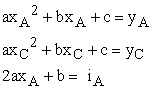Here, the initial slope iA = tgα, where angle α sets the initial direction with the axis OX.

We find consistently a,b,c.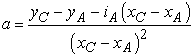(1)

b = iA –2axA and с = yA - axA2 - bxA.

The slope (derivative) at the end of the element is equal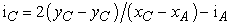(2)

If (yC- yA)/ ( xСА) = iA, then instead of a parabola, we have a straight as special case (iA = iC). For different values of yC we get different shapes (convex, concave, with the vertex inside or outside the element).

Note, if we change yC on Δ (discrete) then final slope iC changes on 2Δ/( xСА).

If at least one parameter of some parabola doesn't satisfy at least one restrictions, then such parabola is ignored. For each of the remaining parabolas we calculate the value of criteria (index of quality approximation). The restrictions on the curvature are reduced to bilateral restrictions on the parameter a, if the slopes (the first derivative of the approximating curve) much smaller than unity, which is the case in the problems of road design. In any case, it is easy to verify all restrictions for any variant of the parabola.

Instead of complete enumeration of options yc, we can calculate the parameters a, b, c minimizing the integral of the square of the difference between the original and the approximating curve in the considered interval. If the restrictions are violated, we correct the value of corresponding parameter and then calculate yC. However, we must add the grid (some nodes) relatively to the resulting point, which is close to the point on the original curve with the same abscissa. So, the use of the best (local !) approximation have no meaning

When we construct the second and all subsequent elements, each point on the vertical1 should be seen as the start. On the first element that was the point A with one possible value of the initial slope, now there are a lot of points, and in each of them there are several values of the initial slope.

If the number of points on each vertical is equal m, then the number of paths that lead from the starting point A to the second vertical is equal m, to the third vertical is equal m2, later m3 and so on.

The part of these paths is unacceptable, but overall their number is growing rapidly.

Due to restrictions we can not take a single point on the vertical as the "system state". It is necessary to add the value of slope (derivative) in it. We may compare only paths converging with the same slopes. It means that the paths which we may compare have a common end point and end slope. Of all such paths we leave only one for further consideration. Its value of total criterion is minimal.

We denote xj, yj-coordinates of the beginning of the j-th element, Lj = xj+1-xj, ij - the slope at the beginning of j-th element.

In accordance with formula for the end slope (2), we may compare variants of j-th elements, which have the same length Lj, if 2(yj′′-yj′) = (ij′-ij′′)Lj. Here yj′, ij′ and yj′′, ij′′ the ordinates and the slopes at the beginning of the j-th element for two its variants, which have the same end point and end slope.

At such rule of rejection the variants their number increases dramatically, resulting in an insuperable computational difficulty even if the lengths of the elements are known. Instead of, we assume that variants which converge at one point are comparable if the difference of their final slopes is small, that is, we introduce the discreteness of the slopes. This means that we use two parameters for each state but the grid for second parameter (slope) is constructed during the solution.

On the second and all subsequent steps, we proceed as follows (Figure 3).

1. We take the point on last considered vertical (on the second step this is 1 vertical), which has the lowest ordinate (point C). Than we construct the allowable parabola to the lowest point of the next vertical (point D). We leave only allowable options for further analysis and for each of them we calculate the total value of the criterion from the beginning of the curve (point A) to the current point D.

2. We take the next point on the left vertical (point E) and we construct the parabola from this point to point D using one of the initial slopes. But in this case we use the following rule of rejection: if the slopes at the end of the parabolas that converge at the current point (D) are not significantly different (|ij′′+1 – i′j+1| < ε), then we leave only option with the lower criterion value.

The value of ε plays the role of the discrete for slopes. It should be chosen so as the maximum deviation which arise due to it on the next element does not exceed the grid discrete Δ.

The concern is that in the selection of the next parabola we want to know the influence of the small variation of the initial slope, which is used to calculate the parameters. a, b, c. Change ij causes a change in these parameters, and as a result we get another parabola, but with the same start and end points. The difference between the ordinates of two such parabolas reaches an extreme value in their center.

Let us find the maximum deviation within the element between the two parabolas, the initial slopes of which differ by ε. Obviously, this deviation does not change if the origin of coordinates will be placed at the starting point of the element. This significantly simplifies the calculation of the parameters.

Thus, хА = 0; yA = 0; b = ij ; c = 0; xC = Lj; yC = yj+1-yj is known and don’t change. For unknown parameter a we have yj+1-yj = aLj2 + ijLj. It follows that. Here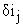- change of the initial slope, and δa consequent change in the parameter a. In the mid-point of the element difference between the ordinates of two parabolas with initial slopes ij andis equal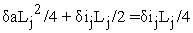.

This difference should not exceed the grid discrete Δ, therefore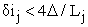.

We take discrete of slope as half ε = 2Δ/ Lmin, where Lmin-minimum length of the element (on axis OX), because the consideration of the elements that are longer 2Lmin has no special meaning. Each of such element can be constructed as a combination of two elements.

3. By turns we consider connection all points of the left vertical with the point D on the right vertical. We reject a part of allowable connecting parabolas using the rule formulated above. Futher we store slopes in the end point D and for each of them total value of the criterion, and the corresponding point on the left vertical.

4. We go to the next point on the right vertical and perform the same action.

As a result, we have "fan" slopes at each point on the right vertical, which are considered in the next step as the initial. Naturally, at the last step it is only one point on the right vertical. It is endpoint B. For endpoint B we find the best point on the one of previous verticals, so total value of the criterion will be minimum. And for this point we have stored a slope and a point on the one of previous vertical and so on.

In other words, in the current state (the point plus the slope) were a lot of incoming paths, but we need in only the best one (totally from point A). Therefore, it is necessary to remember number of the vertical, and state on it, which is the beginning of the last element on optimal path to the current state.

This information allows to restore the entire line using the reverse movement to the point A.

If at the point B the final direction is set, it is necessary to take it into account when calculating the allowable variants of the last parabola.

Now we consider the problem with unknown lengths of the elements. In addition to discrete on vertical Δ and on slopes ε, we introduce the discrete on length of the element λ.

The presence of the discreteness is characteristic for the route design and it is a complicating factor in the use of nonlinear programming. In this case, on the contrary, discreteness simplifies the problem.

Possible length of the first element is in the range from Lmin to 2Lmin with step λ. This means that the start point A (Figure 2) may be connected with the points on not only one but on several verticals (Figure 4).

Each of the possible points on these verticals are considered as the beginning of the second element and so on. "System state" is still a point and slope, but the number of verticals ("process steps") is much greater than with the known lengths of the elements.

Moreover, for storing the connection with the previous state we should store this state (the start point and slope at the beginning of the current element) and additionally number of the vertical to which this start point belongs. A step of the process is not a transition to the next element, but to the next vertical, as one and the same point on vertical may be the endpoint of the few paths, which are composed of a different number of elements.

As before, the total value of criterion ( from point A up to current point) is calculated and stored for each state (point plus slope). We check all restriction and reject the unsuitable variants. The valid paths with a common endpoint, and near values of the final slopes are considered as comparable. In accordance with Bellman’s principle of optimality [13, 14, 15] from all comparable paths in each state we leave for further consideration only one, for which the total value of criterion is minimal. As before, the problem is two-parameter, but a number of paths is significantly greater than for defined lengths of the elements.

The last vertical is spaced from the endpoint B of approximated curve on Lmin. After this vertical will be reached we will consider all valid connections of point B with all points on all verticals, which are spaced from point B on 2Lmin and less. Thus we construct the last element CB (Figure 5).

For all valid variants of the last element CB we calculate the value of criterion and add it to the value of criterion which is stored for point C and each incoming slope. All valid path from all states (point C plus incoming slope) to point B are comparable and we find the optimal variant of the last element and minimal value of the total criterion.

The optimal trajectory is restored by moving in reverse. We use number of the point, the slope and number of the previous vertical, which were stored for connection.

Indeed, for start state (point C plus slope) of the selected variant of the last element we know the start state of the last but one element and so on. Thus, as result we will find the number of elements of the optimal approximating curve and all their parameters.

If the neighboring element beyond of the endpoint B and it slope ifin (Figure 5) are given then at the last step when connecting to the point B we take it into account. From all valid variants of the last element we must consider only those whose slope at the endpoint is equal to the slope of this neighboring element ifin.

If there is no such elements, then we consider as comparable all elements whose slope at the end point deviates from ifin less then on ε.

Briefly algorithm can be represented by the following steps:

0. Constructing the grid variation within the restrictions of type 1 using the discretes λ and Δ.

1. We construct and estimate a set of valid variants of the first element emanating from the starting point. Possible length of the first element is in the range from Lmin to 2Lmin with step λ.

2. At each point of each vertical from 2Lmin+ λ we construct the set of valid variants (incoming fan) and of the variants having similar slopes we choose the best.

Note that unlike the dynamic programming algorithm proposed early in each point is not one but many incoming paths. For each of them we remember the begin of last element (number of the vertical and number of the point on it), slope, and the total value of the criterion.

3. This process continues if the length to the end point is more than Lmin. Otherwise, as the next point considered only the end point. Of all the valid paths which lead to the end point we choose the best.

4. The optimal trajectory is restored by moving in reverse.

### 4. Results

It is known that the practical application of dynamic programming for a large number of states at each step involves dealing with significant computational complexity which dramatically increases with the number of states [7, 8]. This phenomenon is called "curse of dimensionality."

The purpose of experimental calculations was to establish the possibility of practical application of the algorithm for designing the longitudinal profile of new roads on public computers with the search area width 0.5-0.6 m.

Due to the restrictions of type 1 and 2 we cannot perform calculations with a grid with large cells (for example 0.1-0.2m), followed by splitting them into a finer grid depending on the solution obtained. Therefore, we have 25 or more points on each vertical, when width of the search area is 0.5-0.6 m.

With the development of computer programs that implement the algorithm for piecewise parabolic approximation, it was possible to substantially reduce the computation time by putting in order the elements of "incomming fan" at each state.

It was established experimentally that when the number of discrete on each vertical k ≤ 30, the number of slopes on each state m ≤ 60, lmin = 200 m, λ = 10 m and the length of route L ≤ 10000m there are not significant computational difficulties. Such tasks were solved on computer with RAM = 512 Mb, CPU 2.0 GHz. But computational difficulties for this computer became insuperable if k = 70, m = 80, lmin = 200 m, λ = 10 m, L = 10000m.

### 5. Discussion

The proposed algorithm is one element of a multi-stage technology computer-aided design of the longitudinal profile of the roads. This technology is based on a complex optimization methods: nonlinear and dynamic programming. It has clear advantages over the interactive elaboration of design solutions.

The use of complex mathematical models can solve design problems with their relationship and create intelligent CAD. Design of longitudinal profile using optimization algorithms gives a chance for objectively comparison the various plan options and for choosing the most appropriate one. This approach is more promising than the various heuristic algorithms for road routes design.

Computational difficulties with which have to deal, can be overcome in various ways:

1. The use of more powerful computers. But this is not always possible;

2. Decrease in the number of stored parameters for each state. To communicate with the previous state is not necessary to store the slope, enough to remember number of the vertical at the beginning of the element and number of the point on it. But in this case it is necessary to perform additional calculations for restoring the optimal trajectory by reverse moving. For this purpose, we use the formula 2 in reverse order and calculate the slope at the beginning of the current element.

3. On the second stage of projecting involving the road longitudinal profile we don't need in high accuracy of approximation, since we only want to find the number of elements and their approximate position. On the third stage we will get the final decision using non-linear programming. So we may use discrete Δ = 0.02 m instead of 0.01m. Accordingly k = 30 and m = 60 are enough.

4. Using formulae for calculation parabola parameters (1) and end slope (2) we may interrupt the enumeration of the states when some restrictions are not valid. For example if ij+1 < imin (see formulae 2) there is no sense in continuing the search with an increase in ij for the current point.

### 6. Conclusion

To apply dynamic programming it is necessary to identify the key concepts: the "step process", the "system state" and give the rule how to construct a set of states at each step, how to reject unpromising paths leading to the current state, and what must be stored to recover the optimal trajectory.

It is done in this article with respect to the piecewise parabolic approximation of the plane curve given sequence of points. The described algorithm is used in new CAD system. It is for the design of the road routes.

The algorithm is easily generalized to the case when the elements are circles and straight lines. This problem arises in the routing of other linear structures such as pipelines

However the approximation algorithm of discretely defined plane curves with restrictions may find other applications that have nothing to do with designing of routes. In particular for the tasks without restriction, this algorithm may be very simplified and its running time repeatedly reduced.

### Statement of Competing Interest

The author has not competing interests.

### References

  CARD/1. URL: http://www.card-1.com/en/home/.In article  Тоpоmаtic Robur. URL: http:// www.topomatic.ru.In article  Bentley Rail Track. http://www.bentley.com/.In article  C. Parkholup “The system for design of transport highways “Trimble Quantm”, Computer Press, CAD and Grafics, No 3, 2013.In article  V. I. Struchenkov. “Optimization Methods in Applied Problems”, Solon-Press, Moscow, 2009.In article  V.I. Struchenkov “Mathematical Models and Optimization in Line Structure Routing: Survey and Advanced Results”, International Journal Communication, Network and System Sciences. Special Issue: Models and Algorithms for Application, 5, 2012.In article  Nguyen, T.P., Debled-Rennesson, I.: “Decomposition of a curve into arcs and line segments based on dominant point detection. Scandinavian Conference on Image Analysis - SCIA 2011 (2011)".In article  Nguyen, T.P., Debled-Rennesson, I.:” A discrete geometry approach for dominant point detection.” Pattern Recognition 44 (2011), 32-44.In article CrossRef  Horng, J.H.: “An adaptive smoothing approach for _tting digital planar curves with line segments and circular arcs.” Pattern Recognition Letters 24 (2003), 565-577.In article CrossRef  Horng, J.H., Li, J.T. “A dynamic programming approach for _tting digital planar curves with line segments and circular arcs. “ Pattern Recognition Letters 22 (2001), 183-197.In article CrossRef  Tortorella, F., Patraccone, R., Molinara, M.: “A dynamic programming approach for segmenting digital planar curves into line segments and circular arcs” In: ICPR. (2008) 1-4.In article  Kerautret B, Jacques-Olivier Lachaud J-O, and Nguyen, T.P. “Circular arc reconstruction of digital contours with chosen Hausdorff error." Discrete Geometry for Computer Imagery, Nancy: France (2011).In article PubMed  R. Bellman “On the approximation of curves by line segments using dynamic programming”. Communications of the ACM vol. 4, №6, 1961, 284-286.In article  R. Bellman and S. Drejfus, “Applied Dynamic Programming”, Princeton University Press, Princeton, 1962.In article  E.S. Wentzel. “Operations Research: Challenges, principles, metodologiya.” KnoRus, Moscow, 2010.In article